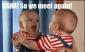# Algebra: Number of layers in the pile of posts

2 posts / 0 new
Celine_101Algebra: Number of layers in the pile of posts

A pile of creosoted poles contains 1275 poles in layers so that the top layer contains one pole and each lower layer contains one more pole than the layer above. How many layers are in the pile? ( SHOW YOUR SOLUTION PLEASE)

Jhun VertThe topmost layer in the pile contains 1 pole, the 2nd layer 2 poles, the third layers 3 poles, and so on. The bottom-most layer, say nth layer, contains n posts.
$1 + 2 + 3 ~ + ... + ~ 5 ~ + ... + ~ n$

The number of poles in these layers of piles is in the form of arithmetic progression (AP) with a common difference d of 1. The first term a1 in AP is 1 (for topmost layer) and the number of terms in AP is the number of layers in the pile. The formula is given by
$S = \frac{1}{2}n[ \, 2a_1 + (n - 1)d \, ]$

$1275 = \frac{1}{2}n[ \, 2(1) + (n - 1)(1) \, ]$

$2550 = 2n + n(n - 1)$

$2550 = 2n + n^2 - n$

$n^2 - n - 2550 = 0$

$(n - 51)(n + 50) = 0$

$n = 51 ~ \text{and} ~ -50$

There are 51 layers in the pile.

• Mathematics inside the configured delimiters is rendered by MathJax. The default math delimiters are $$...$$ and $...$ for displayed mathematics, and $...$ and $...$ for in-line mathematics.